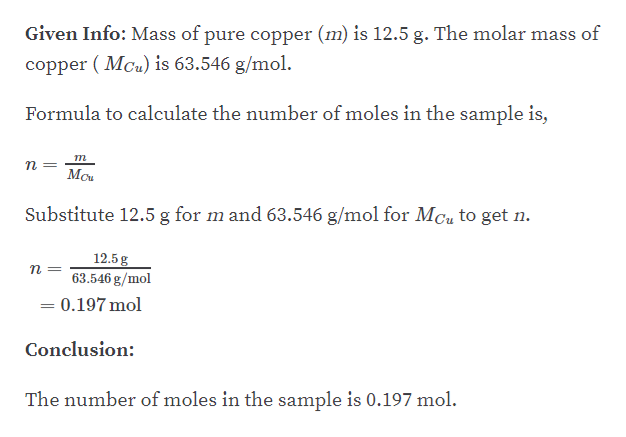# A sample of pure copper has a mass of 12.5 g. Calculate the number of (a) moles in the sample and (b) copper atoms in the sample.

Question
1 views

A sample of pure copper has a mass of 12.5 g. Calculate the number of (a) moles in the sample and (b) copper atoms in the sample.

check_circle

Step 1

Part A:...help_outlineImage TranscriptioncloseGiven Info: Mass of pure copper (m) is 12.5 g. The molar mass of copper ( Mcu) is 63.546 g/mol. Formula to calculate the number of moles in the sample is, т Mou Substitute 12.5 g for m and 63.546 g/mol for MCu to get n. 12.5g 63.546 g/mol = 0.197 mol Conclusion: The number of moles in the sample is 0.197 mol. fullscreen

### Want to see the full answer?

See Solution

#### Want to see this answer and more?

Solutions are written by subject experts who are available 24/7. Questions are typically answered within 1 hour.*

See Solution
*Response times may vary by subject and question.
Tagged in

### Other